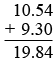SEARCH HOMEMath Central Quandaries & QueriesQuestion from nyheashiacurry, a student: how do you add up decimals?Adding or subtracting decimals is fairly simple. Say for example you want find out the answer for 10.54 + 9.3

Line up the decimal places and make sure each term has the same number of decimal places by add 0s at the end. Working with decimals follows the same rules for addition and subtraction.JaniceMath Central is supported by the University of Regina and The Pacific Institute for the Mathematical Sciences.